# CIE A Level Maths: Mechanics复习笔记4.1.1 Work

### Work

#### What does the term work mean in Mechanics?

• In Mechanics the word work refers to the work done by a force when it causes an object to move
• Mechanical work happens when both a force is applied and the object moves
• A force that is holding an object stationary is not producing any mechanical work
• The object gains energy due to the work done by forces acting in the direction of motion
• The object loses energy due to the work done against resistive forces
• The line of action of a force refers to the point of application of the force and the direction the force was applied in
• Work is a scalar quantity, it has size without direction

#### How do we calculate work done by a force?

• If the point of application of a force of magnitude F N moves an object a distance, d metres in the same direction as the line of action of the force the work done, W  , by the force is
•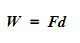If the line of action of the force is at an angle to the direction of motion, then the component of the force in the direction travelled is multiplied by the distance instead
• If the object moves vertically upwards then the work is done against gravity and this calculation becomes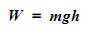• The units for the work done by a force are Newton metres (N m), but it is more common to use Joules
• 1 Joule = 1 N m
• 1 Joule is equal to the amount of work done by a force of 1 Newton moving an object 1 metre along the line of action of the force
• 1 Kilojoule is equal to 1000 Joules (1 kJ = 1000 J)
• If the line of action of the force is different to the direction of motion, start by resolving the force into components parallel and perpendicular to the direction of motion
•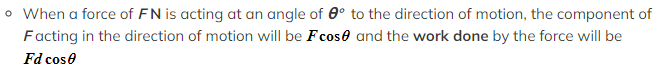The perpendicular component of the force will produce no work
• The net work done on an object will be equal to the work done by the force that move the object forwards against any work done against resistive forces

#### How do we use work done with N2L (F = ma)?

• If the work done by a force is known it can often be used along with N2L to find one of the components in the formula
• Often the object will be moving at constant speed so the acceleration of the object will be zero so the forces acting on the object in the direction of motion will be in equilibrium

STEP 1:  Draw a diagram or add all the forces to the diagram given in the question

STEP 2:  If the line of action of the force is at an angle to the direction of motion, find the component of the force parallel to the direction of motion

STEP 3:  If necessary, resolve the forces acting in the direction of motion to find the magnitude of the force acting on the object

STEP 4:  Use W = Fs  to find the work done by the force on the object

• If the problem involves friction, you may have to resolve perpendicular to the plane to find the value of the normal reaction force, R
• If the force overcomes friction to cause an object to move, it is said to do work done against friction

####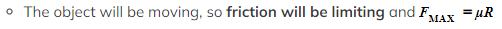Worked Example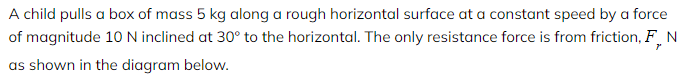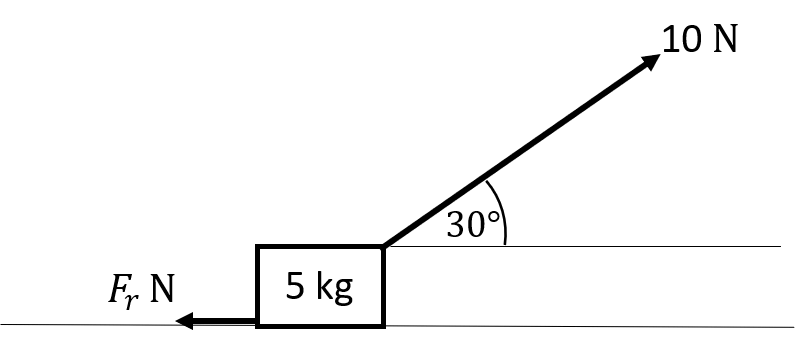Calculate the work done against friction as the child pulls the box 5 metres along the floor.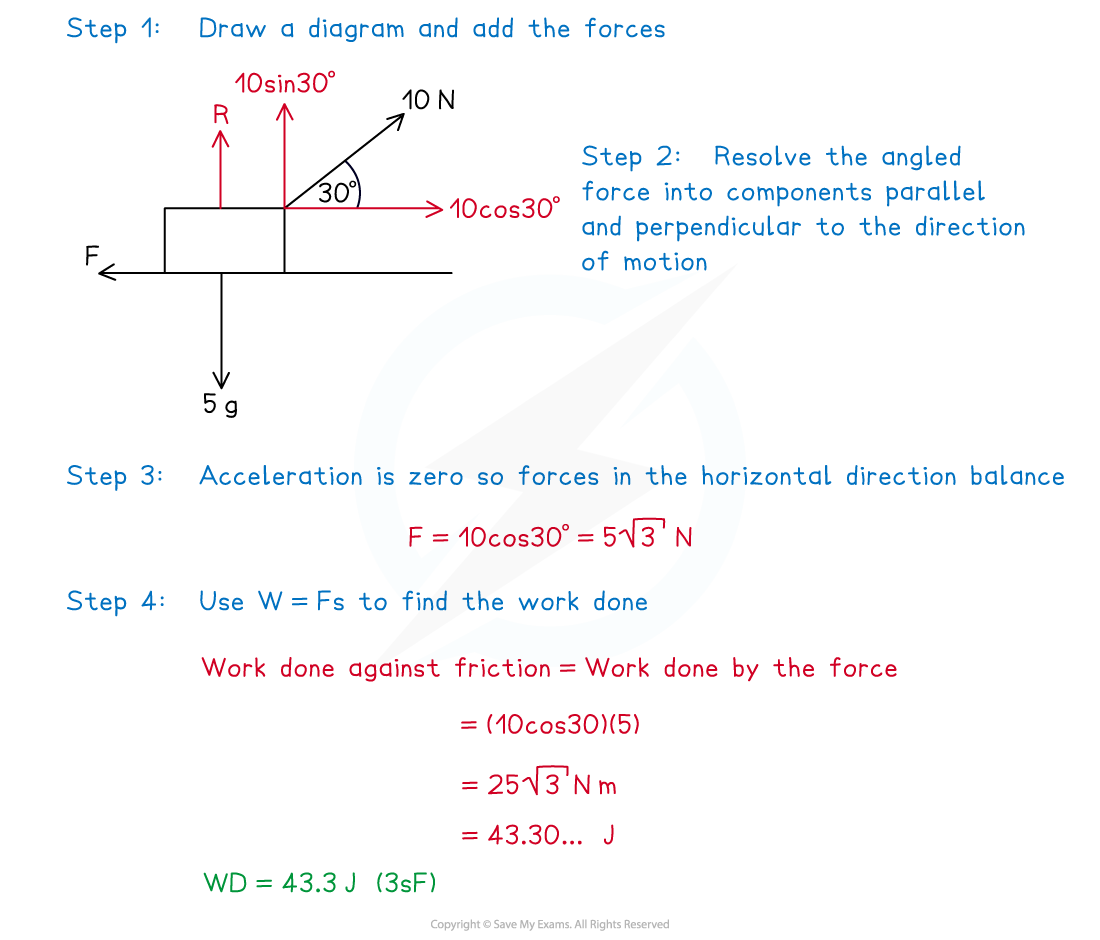#### How do we use work done on an inclined plane?

• The object will be said to travel up the line of greatest slope on an inclined plane
• If the coefficient of friction is involved then the weight will need to be resolved to find the value of the normal reaction force, R
• There is no work done by the normal reaction force as it acts perpendicular to the direction of motion
• The work done against gravity can be found by using right – angled trigonometry to find the change in vertical height of the object

#### Worked Example

A crate of mass 10 kg is pushed 6 metres up a rough ramp inclined at 15° to the horizontal by a force F N.  The crate moves with constant speed along the line of greatest slope. The coefficient of friction between the container and the ramp is 0.2 as shown in the diagram below.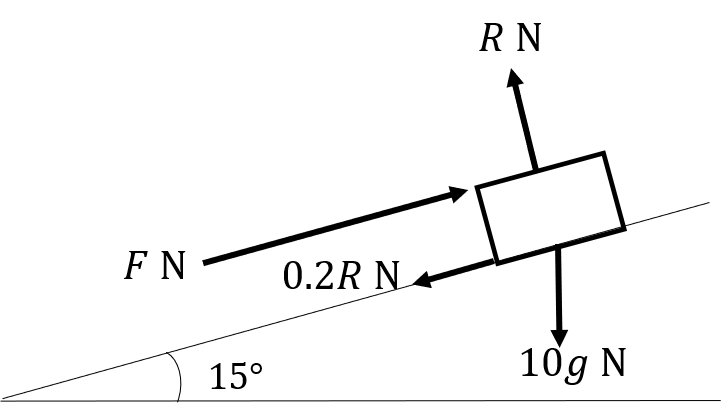(i)Calculate the work done against friction.
(ii)Calculate the work done against gravity.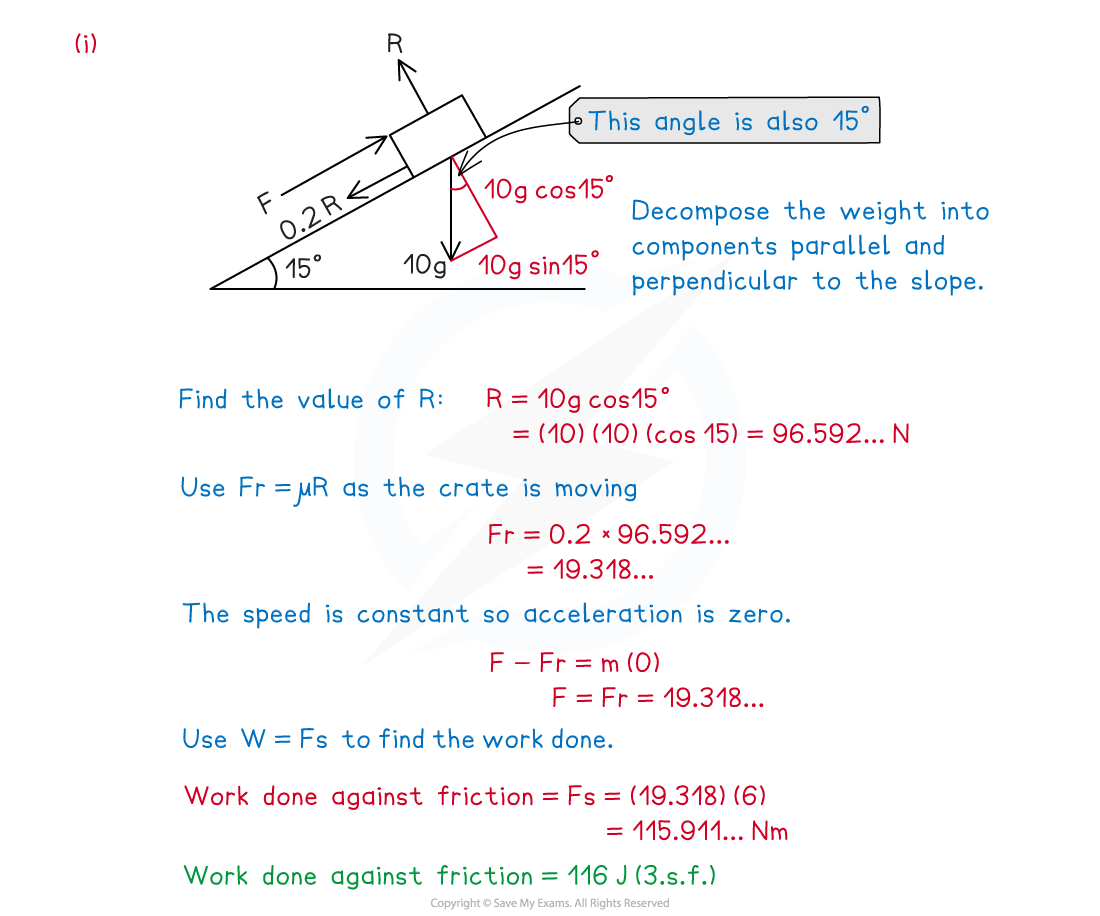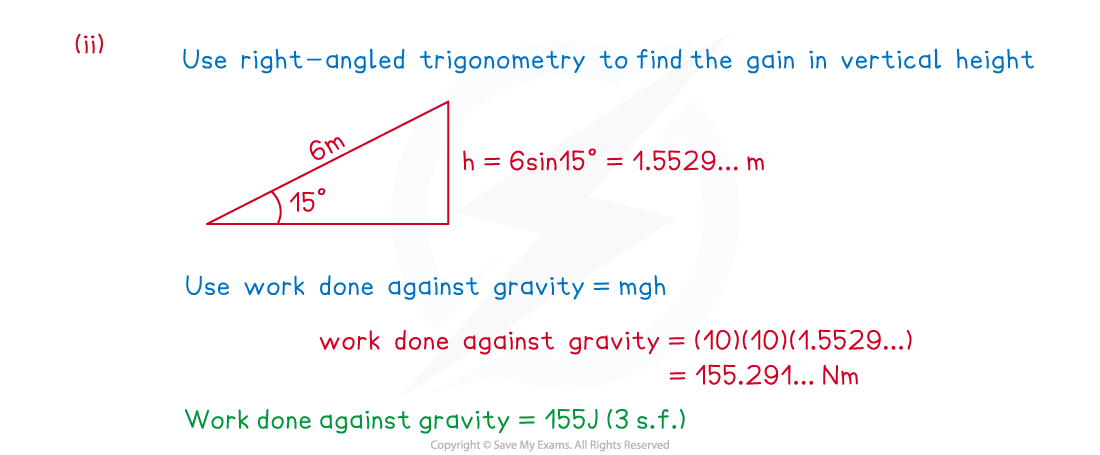#### Exam Tip

Read the question carefully to decide if the point of application of the force is acting in the direction of motion. Always draw a diagram or add to the diagram given in the question. Check to see if there are any resisting forces.